JEE  >  Test: Single Correct MCQs: Laws of Motion | JEE Advanced

# Test: Single Correct MCQs: Laws of Motion | JEE Advanced

Test Description

## 16 Questions MCQ Test Physics For JEE | Test: Single Correct MCQs: Laws of Motion | JEE Advanced

Test: Single Correct MCQs: Laws of Motion | JEE Advanced for JEE 2023 is part of Physics For JEE preparation. The Test: Single Correct MCQs: Laws of Motion | JEE Advanced questions and answers have been prepared according to the JEE exam syllabus.The Test: Single Correct MCQs: Laws of Motion | JEE Advanced MCQs are made for JEE 2023 Exam. Find important definitions, questions, notes, meanings, examples, exercises, MCQs and online tests for Test: Single Correct MCQs: Laws of Motion | JEE Advanced below.
Solutions of Test: Single Correct MCQs: Laws of Motion | JEE Advanced questions in English are available as part of our Physics For JEE for JEE & Test: Single Correct MCQs: Laws of Motion | JEE Advanced solutions in Hindi for Physics For JEE course. Download more important topics, notes, lectures and mock test series for JEE Exam by signing up for free. Attempt Test: Single Correct MCQs: Laws of Motion | JEE Advanced | 16 questions in 30 minutes | Mock test for JEE preparation | Free important questions MCQ to study Physics For JEE for JEE Exam | Download free PDF with solutions
 1 Crore+ students have signed up on EduRev. Have you?
Test: Single Correct MCQs: Laws of Motion | JEE Advanced - Question 1

### A ship of mass 3 × 107 kg initially at rest, is pulled by a force of 5 × 104 N through a distance of 3m. Assuming that the resistance due to water is negligible, the speed of the ship is

Detailed Solution for Test: Single Correct MCQs: Laws of Motion | JEE Advanced - Question 1

F = ma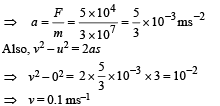Test: Single Correct MCQs: Laws of Motion | JEE Advanced - Question 2

### A block of mass 0.1 kg is held against wall applying horizontal force of 5 N on the block .If coefficient of friction between the block and wallis 0.5 then tbe magnitude of frictional force acting on the block is ?

Test: Single Correct MCQs: Laws of Motion | JEE Advanced - Question 3

### A block of mass 0.1 is held against a wall applying a horizontal force of 5 N on the block. If the  coefficient of friction between the block and the wall is 0.5, the magnitude of the frictional force acting on the block is :

Detailed Solution for Test: Single Correct MCQs: Laws of Motion | JEE Advanced - Question 3

Limiting frictional force,  fl = µsN = 0.5 × 5 = 2.5 N. But force tending to produce relative motion is the weight (W) of the block which is less than fl. Therefore, the frictional force is equal to the weight, the magnitude of the frictional force f has to balance the weight 0.98 N acting downwards.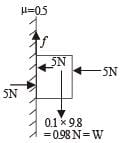Therefore the frictional force = 0.98 N.

Test: Single Correct MCQs: Laws of Motion | JEE Advanced - Question 4

A small block is shot into each of the four tracks as shown below. Each  of the tracks rises to the same height. The speed with which the block enters the track is the same in all cases. At the highest point of the track, the normal reaction is maximum in

Detailed Solution for Test: Single Correct MCQs: Laws of Motion | JEE Advanced - Question 4

Since the body presses the surface with a force N hence according to Newton's third law the surface presses the body with a force N. The other force acting on the body is its weight mg.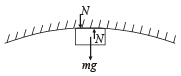For circular motion to take place, a centripetal force is required which is provided by (mg + N).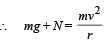where r is the radius of curvature at the top.
If the surface is smooth then on applying conservation of mechanical energy, the velocity of the body is always same at the top most point. Hence, N and r have inverse relationship. From the figure it is clear that r is minimum for first figure, therefore N will be maximum.
Note : If we do not assume the surface to be smooth, we cannot reach to a conclusion.

Test: Single Correct MCQs: Laws of Motion | JEE Advanced - Question 5

An insect crawls up a hemispherical surface very slowly (see fig.). The coefficient of friction between the insect and the surface is 1/3. If the line joining the center of the hemispherical surface to the insect makes an angle a with the vertical, the maximum possible value of a is given by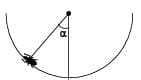Detailed Solution for Test: Single Correct MCQs: Laws of Motion | JEE Advanced - Question 5

KEY CONCEPT : For  the maximum possible value of α, mg sin α will also be maximum and equal to the frictional force.
In this case f is the limiting friction. The two forces acting on the insect are mg and N. Let us resolve mg into two components.
mg cos α balances N.
mg sin α is balanced by the frictional force.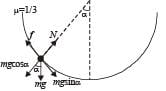∴ N = mg cos α
f = mg sin α
But f = µN = µ mg cos α

∴ µ mg cos α = mg sin α ⇒ cot α =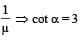Test: Single Correct MCQs: Laws of Motion | JEE Advanced - Question 6

The pulleys and strings shown in the figure are smooth and of negligible mass. For the system to remain in equilibrium, the angle θ should be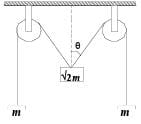Detailed Solution for Test: Single Correct MCQs: Laws of Motion | JEE Advanced - Question 6

The tension in both strings will be same due to symmetry.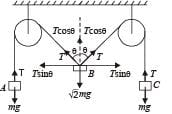For equilibrium in vertical direction for body B we have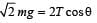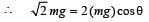[∵ T = mg, (at equilibrium]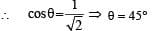Test: Single Correct MCQs: Laws of Motion | JEE Advanced - Question 7

A string of negligible mass going over a clamped pulley of mass m supports a block of mass M as shown in the figure. The force on the pulley by the clamp is given by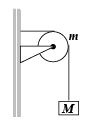Detailed Solution for Test: Single Correct MCQs: Laws of Motion | JEE Advanced - Question 7

At equilibrium T = Mg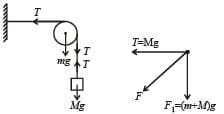F.B.D. of pulley
F1 = (m + M) g
The resultant force on pulley is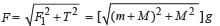Test: Single Correct MCQs: Laws of Motion | JEE Advanced - Question 8

What is the maximum value of the force F such that the block shown in the arrangement, does not move?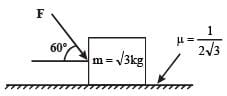Detailed Solution for Test: Single Correct MCQs: Laws of Motion | JEE Advanced - Question 8

The forces acting on the block are shown. Since the block is not moving forward for the maximum force F applied, therefore
F cos 60° = f = µN ... (i) (Horizontal  Direction)
Note : For maximum force F, the frictional force is the limiting friction = µN]
and F sin 60° + mg = N... (ii)
From (i) and (ii)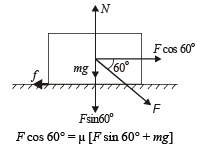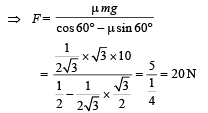Test: Single Correct MCQs: Laws of Motion | JEE Advanced - Question 9

A block P of mass m is placed on a horizontal frictionless plane. A second block of same mass m is placed on it and is connected to a spring of spring constant k, the two blocks are pulled by distance A. Block Q oscillates without slipping.
What is the maximum value of frictional force between the two blocks.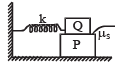Detailed Solution for Test: Single Correct MCQs: Laws of Motion | JEE Advanced - Question 9

Let ω be the angular frequency of the system.
The maximum acceleration of the system,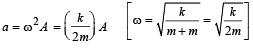The force of friction provides this acceleration.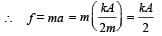Test: Single Correct MCQs: Laws of Motion | JEE Advanced - Question 10

The string between blocks of mass m and 2m is massless and inextensible. The system is suspended by a massless spring as shown. If the string is cut find the magnitudes of accelerations of mass 2m and m (immediately after cutting)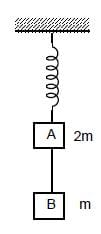Detailed Solution for Test: Single Correct MCQs: Laws of Motion | JEE Advanced - Question 10

In situation 1, the tension T has to hold both the masses 2m and m therefore,
T = 3mg
In situation 2, when the string is cut, the mass m is a freely falling body and its acceleration due to gravity is g.
For mass 2m, just after the string is cut, T remains 3mg because of the extension of string.

∴ 3mg – 2mg = 2m × a    ∴ g/2 = a

Test: Single Correct MCQs: Laws of Motion | JEE Advanced - Question 11

Two particles of mass m each are tied at the ends of a light string of length 2a. The whole system is kept on a frictionless horizontal surface with the string held tight so that each mass is at a distance 'a' from the centre P (as shown in the figure).
Now, the mid-point of the string is pulled vertically upwards with a small but constant force F. As a result, the particles move towards each other on the surface. The magnitude of acceleration, when the separation between them becomes 2x, is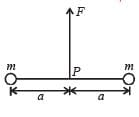Detailed Solution for Test: Single Correct MCQs: Laws of Motion | JEE Advanced - Question 11

The acceleration of mass m is due to the force T cos θ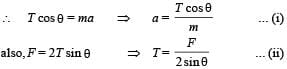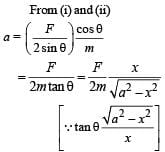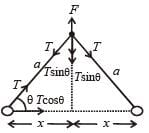Test: Single Correct MCQs: Laws of Motion | JEE Advanced - Question 12

A particle moves in the X-Y plane under the influence of a force such that its linear momentum is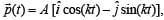where A and k are constants.

The angle between the force and the momentum is

Detailed Solution for Test: Single Correct MCQs: Laws of Motion | JEE Advanced - Question 12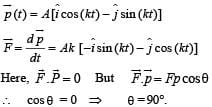Test: Single Correct MCQs: Laws of Motion | JEE Advanced - Question 13

A block of base 10 cm × 10 cm and height 15 cm is kept on an inclined plane. The coefficient of friction between them is √3. The inclination θ of th is inclined plane from the horizontal plane is gradually increased from 0°. Then

Detailed Solution for Test: Single Correct MCQs: Laws of Motion | JEE Advanced - Question 13

For the block to slide, the angle of inclination should be equal to the angle of repose, i.e.,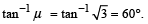Therefore, option (a) is wrong.
For the block to topple, the condition of the block will be as shown in the figure.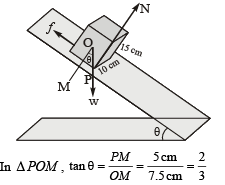For this, θ < 60°. From this we can conclude that the block will topple at lesser angle of inclination. Thus the block will remain at rest on the plane up to a certain anlgle θ and then it will topple.

Test: Single Correct MCQs: Laws of Motion | JEE Advanced - Question 14

A block of mass m is on an inclined plane of angle θ. The coefficient of friction between the block and the plane is m and tan θ > μ. The block is held stationary by applying a force P parallel to the plane. The direction of force pointing up the plane is taken to be positive. As P is varied from P1 = mg(sinθ – μ cosθ) to P2 = mg(sinθ + μ cosθ), the frictional force f versus P graph will look like

Detailed Solution for Test: Single Correct MCQs: Laws of Motion | JEE Advanced - Question 14

As tan θ > μ, the block has a tendency to move down the incline. Therfore a force P is applied upwards along the incline. Here, at equilibrium P + f = mg sin θ   ⇒ f = mg sin θ - P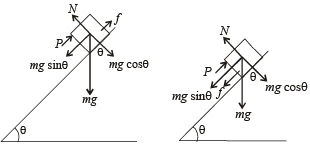Now as P increases, f decreases linearly with respect to P.
When P = mg sin θ, f = 0.
When P is increased further, the block has a tendency to move upwards along the incline.
Therefore the frictional force acts downwards along the incline.
Here, at equilibrium P = f + mg sin θ

∴ f = P – mg sinθ
Now as P increases, f increases linearly w.r.t P.
This is represented by graph (a) .

Test: Single Correct MCQs: Laws of Motion | JEE Advanced - Question 15

A ball of mass (m) 0.5 kg is attached to the end of a string having length (L) 0.5 m. The ball is rotated on a horizontal circular path about vertical axis. The maximum tension that the string can bear is 324 N. The maximum possible value of anguar velocity of ball (in radian/s) is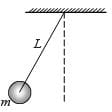Detailed Solution for Test: Single Correct MCQs: Laws of Motion | JEE Advanced - Question 15

Here, the horizontal component of tension provides the necessary centripetal force.
∴ T sin θ = mrω2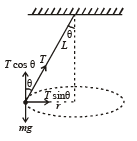From (i) and (ii)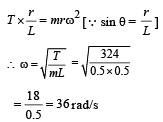Test: Single Correct MCQs: Laws of Motion | JEE Advanced - Question 16

A car sometimes overturns while taking a turn. When it overturns, it is

Detailed Solution for Test: Single Correct MCQs: Laws of Motion | JEE Advanced - Question 16

While turning the maximum allowed speed is:
V max ​ = √μrg ​
where,
μ is coefficient of friction,
r is radius of the turn and
g acceleration due to gravity.
For the inner wheel the radius of the turn is less than the outer wheel and therefore its maximum speed is also less than for the outer wheel. Thus the inner wheel leaves the ground first.

## Physics For JEE

257 videos|633 docs|256 tests
 Use Code STAYHOME200 and get INR 200 additional OFF Use Coupon Code
Information about Test: Single Correct MCQs: Laws of Motion | JEE Advanced Page
In this test you can find the Exam questions for Test: Single Correct MCQs: Laws of Motion | JEE Advanced solved & explained in the simplest way possible. Besides giving Questions and answers for Test: Single Correct MCQs: Laws of Motion | JEE Advanced, EduRev gives you an ample number of Online tests for practice

## Physics For JEE

257 videos|633 docs|256 tests